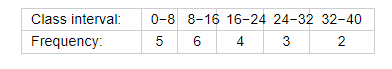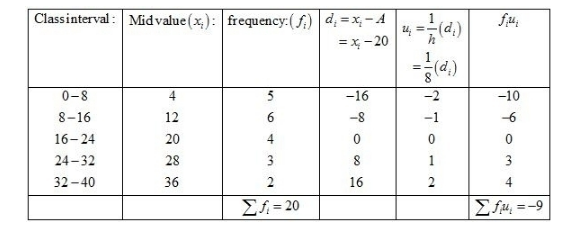# Find the mean of each of the following frequency distributions :

Question:

Find the mean of each of the following frequency distributions :Solution:

Let the assumed mean be A = 20 and h = 8.We know that mean, $\bar{X}=A+h\left(\frac{1}{N} \sum f_{i} u_{i}\right)$

Now, we have $N=\sum f_{i}=20, \sum f_{i} u_{i}=-9, h=8$ and $A=20$.

Putting the values in the above formula, we get

$\bar{X}=A+h\left(\frac{1}{N} \sum f_{i} u_{i}\right)$

$=20+8\left(\frac{1}{20} \times(-9)\right)$

$=20-\frac{72}{20}$

$=20-3.6$

$=16.4$

Hence, the mean is 16.4.